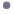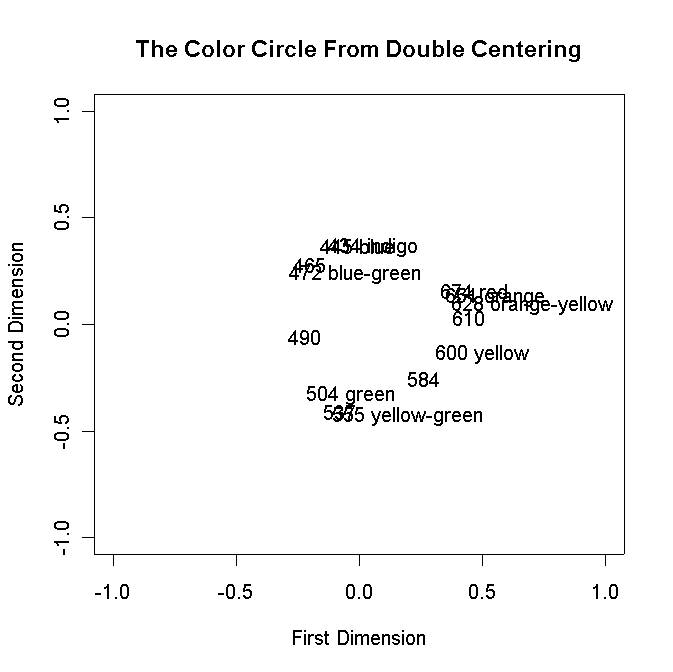This site is an archived version of Voteview.com archived from University of Georgia on May 23, 2017. This point-in-time capture includes all files publicly linked on Voteview.com at that time. We provide access to this content as a service to ensure that past users of Voteview.com have access to historical files. This content will remain online until at least January 1st, 2018. UCLA provides no warranty or guarantee of access to these files.

POLS 6386 MEASUREMENT THEORY
Fifth Assignment
Due 25 February 2003

1. The aim of this problem is to show you how to double-center a matrix in R and extract the eigenvalues and eigenvectors of the matrix.
```
#                                                             The "#" is a comment statement --
# double_center.r -- Double-Center Program                    that is, it tells the R compiler
#                                                             that what follows on this line are
# The Data Are assumed to be Squared Distances -- Data Must   comments, not commands!
#     Be Transformed to Squared Distances Below
#
T <- matrix(scan("D:/R_Files/colors.txt",0),ncol=14,byrow=TRUE)  This reads in the Color Matrix Data --  Note that you have to tell it how many columns!
attach(colornames)                Same as Homework 4
TT <- T                           Copy matrix T into matrix TT
nrow <- length(T[,1])             Counts number of rows
ncol <- length(T[1,])             Counts number of columns
xrow <- NULL                      Initializes Vector
xcol <- NULL                      Initializes Vector
matrixmean <- 0                   Initialize to zero
#
# Transform the Matrix            This transforms the similarities data from a range of
#                                 0 - 100 to a range of 0 - 4 in squared distances
i <- 0
while (i < nrow) {                Note that this loops nrow times because it starts at 0!
i <- i + 1                      Increment the counter or it will loop forever!
j <- 0                          Initialize column index to 0
while (j < ncol) {              Note that this loops ncol times because it starts at 0!
j <- j + 1                   Increment the counter or it will loop forever!
TT[i,j] <- ((100 - T[i,j])/50)**2 Here is the Transformation
}
}
T <- TT                           Copy matrix TT into matrix T
#
# Set Row and Column Vectors to zero
i <- 0                            These two loops simply initialize the vectors to zero
while (i < nrow) {
i <- i + 1
xrow[i] <- 0.0
}
i <- 0
while (i < ncol) {
i <- i + 1
xcol[i] <- 0.0
}
# Compute Row Means and Matrix Mean -- Set TT(,) to Zeroes
i <- 0                            Set counter to zero
while (i < nrow) {                Loop over Rows
i <- i + 1                      Increment the counter or it will loop forever
j <- 0                          Set column counter to zero
while (j < ncol) {              Loop over Columns to get Row Means
j <- j + 1                   Increment the counter or it will loop forever
xrow[i] <- xrow[i]+T[i,j]/ncol Add up elements in the row (add across columns)
matrixmean <- matrixmean + T[i,j]/(nrow*ncol) This gives us the matrix mean
TT[i,j] <- 0                 Initialize matrix TT to zero
}
}
# Compute Column Means
j <- 0                            Set counter to zero
while (j < ncol) {                Loop over Columns
j <- j + 1                      Increment the counter or it will loop forever
i <- 0                          Set row counter to zero
while (i < nrow) {              Loop over Rows to get Column Means
i <- i + 1                   Increment the counter or it will loop forever
xcol[j] <- xcol[j]+T[i,j]/nrow Add up elements in the column (add across rows)
}
}
# Double-Center the Matrix        This Double-Centers the Matrix
i <- 0                            Set counter to zero
while (i < nrow) {                Loop over Rows
i <- i + 1                      Increment the counter or it will loop forever
j <- 0                          Set column counter to zero
while (j < ncol) {              Loop over Columns
j <- j + 1                   Increment the counter or it will loop forever
TT[i,j] <- (T[i,j]-xrow[i]-xcol[j]+matrixmean)/(-2) TT is the Double-Centered Matrix
}
}                                 End of Double-Center Code
ev <- eigen(TT)                   Compute the Eigenvalues and Eigenvectors
DIMNEW.1 <- ev\$vec[,1]            Put the First Eigenvector in DIMNEW.1
DIMNEW.2 <- ev\$vec[,2]            Put the Second Eigenvector in DIMNEW.2
plot(DIMNEW.1,DIMNEW.2,type="n",main="The Color Circle From Double Centering",
xlab="First Dimension",ylab="Second Dimension",
xlim=c(-1.0,1.0),ylim=c(-1.0,1.0))                   Plot Commands
```
Download the R program and two example files below and place them in the same directory on your disk. Note that you will have to use Epsilon to change the path statements in double_center.r.R Program to Perform Double-CenteringEkman Color Similarities MatrixColor Coordinates With Row Names Used in Homework 4

Run double_center.r and you should see:1. Turn in the Graph.

2. In R type:

eigenvalue <- abs(ev\$val)
eigenvalue

and you should see:
```
 7.928536e+00 5.197332e+00 1.763696e+00 1.495726e+00 6.338461e-01
 4.120299e-01 1.660689e-01 1.266656e-01 7.386280e-02 1.666023e-02
 5.380350e-03 9.296853e-15 1.069314e-01 1.897294e-01```
These are the eigenvalues of the double-centered matrix (technically, the absolute values of the eigenvalues). Paste these into Excel and make a plot like that shown in Home Work Number 3, problem 2.b. Turn in this plot with the appropriate title (e.g., "Eigenvalues of Double-Centered Color Data").

3. We are going to test Shepard's Universal Law by assuming that the color data are actually generated by an exponential loss function. To do this:

• Make a copy of double_center.r -- double_center_2.r.

• Bring double_center_2.r up in Epsilon.

• Change the line "TT <- T" that comes right after the line "attach(colornames)" to:
```
TT <- ifelse(T <= 5,5,T)   If T[i,j] £ 5 set T[i,j] = 5,
else if T[i,j] > 5 leave as is (replace with itself)```
• Right below this line insert the line:

T <- TT

These two commands replace any entries in "colors.txt" that are less than 5 with 5 because the natural log of zero is negative infinity!

• Replace the line "TT[i,j] <- ((100 - T[i,j])/50)**2" with:

TT[i,j] <- ((-1.0)*log(T[i,j]/100))**2

Repeat parts (a) and (b) with the plots appropriately titled.

2. In this problem we are going to use KYST to replicate the analysis done by Herbert F. Weisberg and Jerrold G. Rusk in their 1970 paper "Dimensions of Candidate Evaluation," American Political Science Review, 64 (Dec. 1970): 1167-1185. Download the following data file:1968 Election Data

The 1968 Election Data file contains some variables besides the thermometer scores that we will use in future homeworks. The variables are:
```
idno           respondent id number
partyid        strength of party id -- 0 to 6
income         raw income category
incomeq        income quintile -- 1 to 5
race           0 = white, 1 = black
sex            0 = man, 1 = woman
south          0 = north, 1 = south
education      1=HS, 2=SC, 3=College
age            age in years
uulbj          lbj position urban unrest
uuhhh          humphrey pos urban unrest
uunixon        nixon position urban unrest
uuwallace      wallace pos urban unrest
uuself         self placement urban unrest
vnmlbj         lbj pos vietnam
vnmhhh         hhh pos vietnam
vnmnixon       nixon pos vietnam
vnmwallace     wallace pos vietnam
vnmself        self placement vietnam
voted          1=voted, 5=did not vote
votedfor       who voted for -- 1 = humphrey, 2= nixon, 3=wallace
wallace        wallace therm
humphrey       humphrey thermometer
nixon          nixon thermometer
mccarthy       mccarthy thermometer
reagan         reagan thermometer
rockefeller    rockefeller thermometer
lbj            lbj thermometer
romney         romney thermometer
kennedy        robert kennedy thermometer
muskie         muskie thermometer
agnew          agnew thermometer
lemay          "bombs away with Curtis LeMay" thermometer
```
1. Make a copy of OLS68B.DAT -- therm68.txt -- and use Epsilon to remove all the variables except the 12 Thermometer Scores. Turn in the Epsilon macro you used to create therm68.txt.

2. Read the data into R with the command (be sure you use the appropriate path statement!):

T <- matrix(scan("D:/R_Files/therm68.txt",0),ncol=12,byrow=TRUE)

The range of the 1968 Feeling Thermometers was 0 to 97 -- 98 and 99 were used as missing values. You need to tell R that 98 and 99 are missing. To do this, use the following command:
```TT <- ifelse(T==98 | T==99,NA,T)  If T[i,j] = 98 or 99 set TT[i,j] = NA (missing data)
else if set TT[i,j] = T[i,j]```
To compute the correlation matrix use the command:

R <- cor(TT,use="pairwise.complete.obs")

The "pairwise.complete.obs" tells R to throw away missing data pair-wise, not list-wise (that is, the whole row of data!). Now type:

R

and you should something like this:
```
[,1]       [,2]        [,3]   ....etc
[1,]  1.00000000 -0.3108400  0.01690949   ....etc
.... etc etc etc ...```
You need this correlation matrix to duplicate what Weisberg and Rusk did with KYST. Use Epsilon to copy the correlation matrix into your KYST control card file. Note that you will have to reassemble the matrix using Epsilon and note that because of the pecularities of R, the second column of the matrix has one less digit than the other columns!!! You will have to stick a zero at the end of each correlation in the second column to get everything to work. Your KYST control card file should look something like this:
```
TORSCA
PRE-ITERATIONS=3
DIMMAX=3,DIMMIN=1
PRINT HISTORY,PRINT DISTANCES
COORDINATES=ROTATE
ITERATIONS=50
REGRESSION=DESCENDING
DATA,LOWERHALFMATRIX,DIAGONAL=PRESENT,CUTOFF=-2.00
1968 FEELING THERMOMETER CORRELATION MATRIX
12  1  1
(14X,12F12.8)
Wallace        1.00000000 -0.31084000  0.01690949 ... etc etc
...etc etc ...
COMPUTE
STOP```
Report the Stress Values for 1, 2, and 3 dimensions and use R to graph the results in two dimensions.

3. Produce a Shepard Diagram for the two dimensional solution (the horizontal axis is the actual correlation data -- "DATA" in the KYST output file -- and the vertical axis is the estimated distances -- the "DIST" column in the KYST output file).

4. Make a plot of the first two eigenvectors of the correlation matrix using R (see problem 1 for how to do this -- be certain everything is properly labeled!).

5. Make a plot of the eigenvalues of the correlation matrix (see problem 1).

6. In R create the following 12 by 12 matrices (the t(ev\$vec) is the transposed 12 by 12 matrix of eigenvectors and the symbol "%*%" is the matrix multiplication command):

hello <- diag(12)
ZZ <- t(ev\$vec) %*% ev\$vec

Are these two matrices -- hello and ZZ -- the same?

7. In R create the following matrices:

diag(hello) <- ev\$val
RWORK <- ev\$vec %*% hello %*% t(ev\$vec)

What is RWORK? Be Specific!

3. In this problem we are going to treat the morse code dataset as an unfolding problem. Download the KYST control card file:Morse Code Data in Unfolding Format

1. Run KYST and note that you will end up with 72 points! Point number 1 is "A" and point number 37 is also "A"! (Ignore the tokens in KYST!! They will be off on this problem because there is no "0" -- zero!) Graph the 72 points with the appropriate labels and appropriate titles in R.

2. Produce a Shepard Diagram for the two dimensional solution (the horizontal axis is the actual correlation data -- "DATA" in the KYST output file -- and the vertical axis is the estimated distances -- the "DIST" column in the KYST output file).